# Computer Science : Recursion

## Example Questions

← Previous 1

### Example Question #201 : Computer Science

int lairotcaf (int n) {

if (n <= 1){

return 1

}

temp = n * lairotcaf(n-1)

return temp;

}

int num;

cin >> num;



if (num >= 0){

lairotcaf(n)

} else {

cout << "must use number greater or equal to zero"< endl;

}

What is returned by lairotcaf(5)?Explanation:

In recursion, a value is calculated in reverse until a point at which an answer is defined. From that point, the definition is used to calculate forward, evaluating the other definitions which rely upon that base condition.

Recursive functions must have a test that will halt recursion. If the current state of the recursive function matches the base condition, the recursion should stop. Otherwise, recursion should continue.

In case you did not notice from the backwards-naming scheme, this is a definition for the factorial function. The function will not be called if a negative number is passed. If 0 or 1 is passed, 1 is returned. 0 or 1 is the base condition. If the number is greater than one, a chain of calls to lairotcaf() will initiate until a base condition is reached.

Once lairotcaf(5) is expanded, we have:### Example Question #1 : Recursion

Consider the following code:

public static void main(String[] args) {

System.out.println(equation(8));

}

public static int equation(int a) {

if(a <= 5) {

return 12;

}

return equation(a-2) * equation(a-1);

}

What is the output for the code above?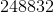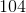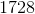Explanation:

The function equation is a recursive method, meaning that it calls itself.  The easiest way to trace a recursive method is to first pay heed to its stopping case.  For this, it is:

if(a <= 5) {

return 12;

}

In this case, it returns 12 for any values less that or equal to 5.  Now, begin your trace:

equation(8) = equation(6) * equation(7)

equation(7) = equation(5) * equation(6)

equation(6) = equation(4) * equation(5)

Therefore, equation(6) is:

12 * 12 or 144

Thus, we know:

equation(7) = 12 * 144 = 1728

And, equation(8) = 144 * 1728 = 248832

### Example Question #1 : Recursion

Which of the following is a recursive factorial function?

Recall that an example of a factorial is: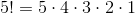None of the others

public long factorial(long a) {

long ret = 1;

for(int i = 2; i < a; i++) {

ret *= i;

}

return ret;

}

public long factorial(long a) {

a * a-1 * a-2 * a-3 * a-4;

}

public long factorial(long a) {

return a * factorial(a-1) * factorial(a-2);

}

public long factorial(long a) {

if(a <= 1) {

return 1;

}

return a * factorial(a-1);

}

public long factorial(long a) {

if(a <= 1) {

return 1;

}

return a * factorial(a-1);

}

Explanation:

Recall that a recursive function calls itself.  Therefore, you can eliminate several of the answers just by the fact that they are not recursive in nature.  (Indeed the one with a loop is a correct computation.)  Now, you can think of a factorial like this: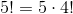And then...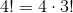And so forth.

Thus, you have a stopping case at 1, which is equal to 1.  For any other value, you will return a recursive value, namely the parameter a and then the factorial method called with a-1.

### Example Question #3 : Recursion

Consider the following code:

public static void main(String[] args) {

     System.out.println(foo("This is my favorite: Yay for programming!!!"));

}



public static String foo(String s) {

     if(!s.equals("")) {

          char c = s.charAt(0);

          if(c >= 'A' && c <= 'Z') {

               return Character.toLowerCase(c) + foo(s.substring(1));

          } else if (c >= 'a' && c <= 'z'){

               return Character.toUpperCase(c) + foo(s.substring(1));

          }

          return foo(s.substring(1));

     }

     return "";

}

What is the output for the main function above?

tHIS IS MY FAVORITE: yAY FOR PROGRAMMING!!!

tHIS IS MYFAVORITE

ThIs iS mY fAvOriTe

tHISISMYFAVORITEyAYFORPROGRAMMING

ThIs iS mY fAvOriTe: YaY fOr PrOgRaMmIng!!!

tHISISMYFAVORITEyAYFORPROGRAMMING

Explanation:

The foo method is clearly recursive. Therefore, let's look at our conditions:

If s is "", then the code reaches the base case (at the very end): return "";

Now, for other strings, there are two cases embedded:

c >= 'A' && c <= 'Z'     and     c >= 'a' && c <= 'z'

Notice that c is the first character of the string. So, for these two cases, if the first character is upper case, the function makes it lower, and if it is lower, it makes it upper case. Then, it makes a recursive call to itself, using the remainder of the string after this first character. If neither of those are reached, it just does a call to itself, ignoring the first character. Therefore, this code will "flip" any cases of alphabetic characters but will ignore all other characters. That is why the correct answer is the compressed-looking string: tHISISMYFAVORITEyAYFORPROGRAMMING

### Example Question #4 : Recursion

Which of the following is a recursive method for summing the contents of a list of integers? (Choose the best answer.)

public static int val(List ints) {

    int ret = 0;

    for(int i = 0; i < ints.size(); i++) {

        ret += ints.get(i);

    }

    return ret;

}

public static int val(List ints) {

     if(ints.size() == 0) {

          return 0;

     } else {

          return ints.remove(0) + val(ints);

     }

}

public int val(List ints) {

     return ints.get(0) + val(ints - 1);

}

public static int val(List ints) {

     if(ints.size() == 0) {

          return 0;

     } else {

          return ints.get(0) + val(ints.subList(1, ints.size()));

     }

}

public static int val(List ints) {

     return ints.get(0) + val(ints.subList(1, ints.size()));

}

public static int val(List ints) {

     if(ints.size() == 0) {

          return 0;

     } else {

          return ints.get(0) + val(ints.subList(1, ints.size()));

     }

}

Explanation:

Recall that a recursive method is one that calls itself, slowly working toward a "base case" at which it terminates. Therefore, you can immediately eliminate the option that has an iterative loop. This is not recursion.

Next, however, there are two methods that have return values:

1. return ints.get(0) + val(ints.subList(1, ints.size()));
2. return ints.get(0) + val(ints - 1);

Neither of these will work, for they never terminate. (Well, they terminate, but only with an error!) Also, the second is nonsensical anyway—you cannot subtract 1 from a list as a whole!

Finally, consider the option with a recursive return value of:

return ints.remove(0) + val(ints);

This actually will add the items correctly. However, by removing items from the list, you will thus destroy the list in the course of adding them! Therefore, the remaining option is the best one for this question:

public static int val(List ints) {

     if(ints.size() == 0) {

          return 0;

     } else {

          return ints.get(0) + val(ints.subList(1, ints.size()));

     }

}

### Example Question #1 : Recursion

public static int foo(int a, int b) {

if(b <= 1 || b <= a) {

return 1;

}

return (b - a) * foo(a,b-1);

}

Based on the code above, what is the value of the following function call:

foo(5,9);

24

18

16

32

36

24

Explanation:

The function foo is recursive, meaning that it calls itself.  Therefore, you should start by looking for its termination point.  Based on the definition above, it terminates when either b <= 1 or b <= a.  In these two cases, it will return 1.

Now, let us do our trace of the calls:

foo(5,9) = (9-5) * foo(5,8) = 4 * foo(5,8)

foo(5,8) = (8-5) * foo(5,7) = 3 * foo(5,7)

foo(5,7) = (7-5) * foo(5,6) = 2 * foo(5,6)

foo(5,6) = (6-5) * foo(5,5) = 1 * foo(5,5)

foo(5,5) = 1

Therefore, carefully tracing back up, we get:

foo(5,9) = 4 * 3 * 2 * 1 * 1 = 24

### Example Question #6 : Recursion

public static int foo(int a) {

if(a <= 3) {

return 1;

}

return a * foo(a - 3) * foo(a - 4);

}

What is the value of the following call to the function defined above:

foo(8)

144

224

160

140

240

160

Explanation:

The foo method is recursive, so you will need to find the stopping case first.  This happens when a <= 3.  At that time, the method returns 1.  Now, based on this fact, we can begin to trace the method:

foo(8) = 8 * foo(5) * foo(4)

foo(5) = 5 * foo(3) * foo(1)

foo(4) = 4 * foo(1) * foo(0)

foo(0) = foo(1) = foo(3) = 1

Thus, we know:

foo(8) = 8 * 5 * 1 * 1 * 4 * 1 * 1 = 160

### Example Question #2 : Recursion

public void draw() {
recurs(11);
}
void recurs(int count){
if (count == 0)
return;
else {
System.out.print(count + " ");
int recount = count - 2;
recurs(recount);
return;
}
}

What does the code print?

9 7 5 3 1

11 9 7 5 3 1

Error, infinite loop.

9 7 5 3 1 -1

11 9 7 5 3 1 -1

Error, infinite loop.

Explanation:

This creates an infinite loop because the condition to end the loop is never reached. Since count is never equal to 0, count continues to be entered into recurs over and over with no end.

### Example Question #3 : Recursion

public void draw() {
recurs(10);
}
void recurs(int count){
if (count == 0)
return;
else {
System.out.print(count + " ");
int recount = count - 2;
recurs(recount);
return;
}
}

What is the output of the code?

10 8 6 4 2

8 6 4 2 0

8 6 4 2

error, infinite loop

10 8 6 4 2 0

10 8 6 4 2

Explanation:

The number 10 is inputed into recurs and prints count,subtracts two from count, then rentered into recurs.

Thus, the first iteration gives, 10.

The second iteration gives as, 10, 8.

It is written this way because it places 8 after the value from the first iteration.

Continuing in this fashion we get,

10 8 6 4 2.

When count reaches 0, nothing is printed.

### Example Question #4 : Recursion

What does this factorial code snippet return after calling run()?

public void run() {

int n = 2;

n = factorial(n);

n = n + factorial(n);

System.out.println(n);

}

public int factorial(int n) {

if (n==1) {

return 1;

}

else {

return n * factorial(n - 1);

}

}

2 will be printed out

6 will be printed out

3 will be printed out

4 will be printed out

4 will be printed out

Explanation:

4 will be printed out because factorial(2) = 2. Adding factorial(2) + 2 = 4. This can also be written as 2! + 2! = 4.

n is 2 when first assigned

n is then assigned to factorial(2) which is 2

n is then assigned n (which is 2) plus factorial(2) which is 2 so 2+2 = 4

n is 4 when it is printed out

← Previous 1